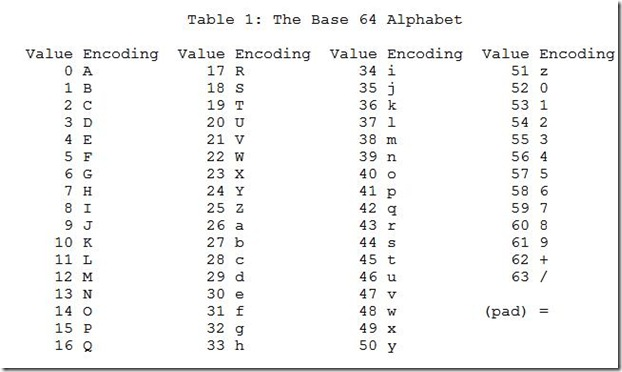# Encoding¶

## What is Encoding?¶

To get started, "Encoding is the process of changing data representation".

Rather, may be we should check this out :

In computer terminology, encoding is the process of applying a specific code, such as letters, symbols and numbers, to data for conversion into an equivalent cipher. The most common example would be changing "abc" to "ABC", the lower-to-upper encoding.

That was pretty basic! Similarly, ASCII (American Standard Code for Information Interchange) is the most common encoding format for text files, be it on your computer or the internet. In an ASCII file, each alphanumeric, digit or special character is represented by an unique decimal number (from 0 to 127). For example, the character 'A' is given code 65, 'B' 66 and so on.

ASCII Table

You can see the complete reference by typing the following in your linux terminal.

\$ man ascii


In addition to just alphabets and digits, ASCII also accounts for non-printable characters (such as newline, backspace etc). So a string, "Hello" can be converted to ASCII as "72 101 108 108 111". This is how computers process information.

In addition to just alphabets and digits, ASCII also accounts for non-printable characters (such as newline, backspace etc). So a string, "Hello" can be converted to ASCII as "72 101 108 108 111". This is how computers process information.

### Number Systems¶

• Binary
• Octal
• Decimal

Decimal is the number system used around the world. But if you peep into Mathematical Computing, we have many number systems. The most prevalent is Binary, which uses just 1's and 0's to represent data [Thus it is a base 2 number system]. Next we have Octal number system with base 8. Here, we use 8 digits to represent data, from 0,1,2 to 7. We also have hexadecimal, another commonly used system. Hexadecimal in fact means a base of 16. Since we only have 10 digits, we also use characters from A to F. So hex characters include 1,2,..,9,A,B,C,D,E and F, making a total of 16 digits. It is possible to convert data from one system to another, but that is beyond the scope of this tutorial.

## Hex Encoding¶

Hex encoding is the process of changing data into hexadecimal representation. Having said that, Hexadecimal numerals are widely used by computer system designers and programmers, as they provide a more human-friendly representation of binary values. You can also try converting decimals and Strings to hex. Each hexadecimal character can be expanded into binary digits (A nibble). And it implies that a byte of data can be represented using two hex chars. Isn't that cool?

Note on Prefixes

In order to differentiate between the representations, we have different prefixes added to the data. \x or 0x is the generally accepted prefix added to hexadecimal string.

### A quick Example:¶

• 0000 of binary will convert to '0' of hex.
• 0001 of binary will convert to '1' of hex. Similarly, 'f' of hex will be 1111 of binary.

Now if we take 8 bits at a time, * 0x00 is 0b00000000, * 0x01 is 0b00000001, and so and so forth till * 0x0f is 0b00001111 (The Decimal equivalent is 15). When we move further, * 0x10 is 0b00010000 (Decimal 16) till * 0xff which is 0b11111111 (Decimal 255).

### The Encoding part¶

Recollect that each character is assigned a decimal equivalent in ASCII [from 0 to 127]. If we try to map it together, it turns out that each of the decimal equivalent can then be converted into hex. That's it! The character 'A' has decimal value of 65, which converts to 0x41 in hex.

So next time we say 0x68656c6c6f, be sure to convert it into ASCII. If you are too lazy (its common among hackers,) it's just "Hello" !

### Python Implementation¶

Python2 vs Python3

In Python 2,implicit string type is ASCII , but in Python 3.x implicit str type is Unicode . So if you are using Python3+, an easy way to work with encoding is to use the codecs module. We shall give the code to encode/decode in python3 additionally. Make sure to type 'import codecs' in the beginning of your code.

Implementing Hex encoding in python is pretty simple. In your python shell, a ''.encode('hex') will convert any string inside the quotes to hex string.

print "This_is_cool!".encode('hex')

546869735f69735f636f6f6c21

print(codecs.encode(b"This_is_cool!",'hex')

b'546869735f69735f636f6f6c21'


To decode a hex string, just change it to ''.decode('hex').

print "546869735f69735f636f6f6c21".decode('hex')

This_is_cool!

print(codecs.decode(b"546869735f69735f636f6f6c21",'hex'))

b'This_is_cool!'


## Base64 Encoding¶

As mentioned, Hex had only 16 characters. But this one is still awesome. Meet the Base64, with 64 characters. Base64 encoding takes three bytes, each consisting of 8 bits. The following is the character set for Base64 - 1. [a-z] - 26 characters 2. [A-Z] - 26 characters 3. [0-9] - 10 characters 4. [+] - 1 character 5. [/] - 1 character Now that if you count, it will add up to 64. It also have '=' character, which is solely used for padding purposes.### Encoding¶

The process is really simple. Write down the binary of the message, taking groups of 6 in one block. Now compare each block with the binary or decimal value of the corresponding character in the Base64 Chart. Join the characters, and that's it. You're done!

Always remember, your Base64 string length should be a multiple of 3. If not, you must add '=' character at the end until it's a multiple. Padding is necessarity required for Base64 and it might save you from Padding errors.

Pro Tips

And if you happen to see '=' at the end of the string, don't hesitate, try a Base64 decode!

### Python Implementation¶

To encode data to base64, we have the following python function.

print "This_is_cool!".encode('base64')

VGhpc19pc19jb29sIQ==

print(codecs.encode(b"This_is_cool!",'base64'))

b'VGhpc19pc19jb29sIQ==\n'


Similar is the decode function for base64.

print "VGhpc19pc19jb29sIQ==".decode('base64')

This_is_cool!

print(codecs.decode(b"VGhpc19pc19jb29sIQ==\n",'base64'))

b'This_is_cool!'